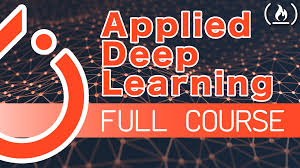# Applied Deep Learning with PyTorch - Full CourseIn this course you will learn the key concepts behind deep learning and how to apply the concepts to a real-life project using PyTorch and Python.

In this course you will learn the key concepts behind deep learning and how to apply the concepts to a real-life project using PyTorch and Python.

You'll learn the following: ⌨️ RNNs and LSTMs ⌨️ Sequence Modeling ⌨️ PyTorch ⌨️ Building a Chatbot in PyTorch

⭐️Requirements ⭐️ ⌨️ Some Basic High School Mathematics ⌨️ Some Basic Programming Knowledge ⌨️ Some basic Knowledge about Neural Networks

⭐️Contents ⭐️ ⌨️ (0:00:08) Recurrent Nerual Networks - RNNs and LSTMs ⌨️ (0:35:54) Sequence-To-Sequence Models ⌨️ (0:44:31) Attention Mechanisms ⌨️ (0:57:17) Introduction to PyTorch ⌨️ (1:10:02) PyTorch Tensors ⌨️ (1:28:03) Chatbot: Processing the Dataset ⌨️ (2:48:12) Chatbot: Data Preperation ⌨️ (3:33:26) Chatbot: Building the Model ⌨️ (4:53:42) Chatbot: Training the Model

## PyTorch for Deep Learning | Data Science | Machine Learning | Python

PyTorch for Deep Learning | Data Science | Machine Learning | Python. PyTorch is a library in Python which provides tools to build deep learning models. What python does for programming PyTorch does for deep learning. Python is a very flexible language for programming and just like python, the PyTorch library provides flexible tools for deep learning.

## Deep Learning Tutorial with Python | Machine Learning with Neural Networks

In this video, Deep Learning Tutorial with Python | Machine Learning with Neural Networks Explained, Frank Kane helps de-mystify the world of deep learning and artificial neural networks with Python!

## Machine Learning, Data Science and Deep Learning with Python

Complete hands-on Machine Learning tutorial with Data Science, Tensorflow, Artificial Intelligence, and Neural Networks. Introducing Tensorflow, Using Tensorflow, Introducing Keras, Using Keras, Convolutional Neural Networks (CNNs), Recurrent Neural Networks (RNNs), Learning Deep Learning, Machine Learning with Neural Networks, Deep Learning Tutorial with Python

## What is Supervised Machine Learning

What is neuron analysis of a machine? Learn machine learning by designing Robotics algorithm. Click here for best machine learning course models with AI

## Python For Machine Learning | Machine Learning With Python

Python For Machine Learning | Machine Learning With Python, you will be working on an end-to-end case study to understand different stages in the Machine Learning (ML) life cycle. This will deal with 'data manipulation' with pandas and 'data visualization' with seaborn. After this an ML model will be built on the dataset to get predictions. You will learn about the basics of scikit-learn library to implement the machine learning algorithm.